## How to Calculate and Compare Watt Hours to Amp Hours

Upgrading your RV or boat’s electrical system with solar solutions can be daunting if you don’t understand the basics of measurement, energy and storage. Let’s see if we can simplify it by translating watt-hours to amp-hours.

### What is a Watt-Hour?

You can use the watt-hour to determine how much energy something uses if you know how many watts it takes and how long it’s been running.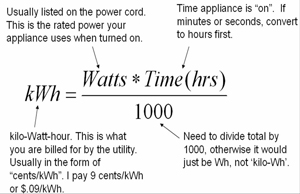From this, we know that one watt-hour is the same as 3600 joules (3.600 x 103 J). So if you want to convert watt-hours to joules, you just have to multiply by 3.600 x 103. And if you have joules and want to convert them to watt-hours, you multiply by 2.778 x 10-4.

Usually, energy is equal to power multiplied by time. This formula lets us calculate the energy in watt-hours:

E = Pt

If we plug in the numbers, we get:

E = 60 x 3 = 180 Wh

Remember that power (P) and time (t) has to be specified in watts and hours, respectively. Otherwise, you’ll need to convert before calculating energy in watt-hours.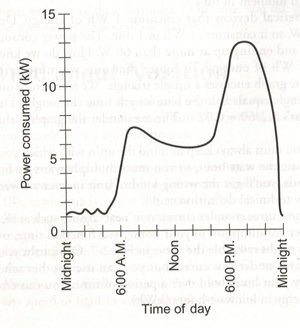##### The graph above depicts the power consumed by a hypothetical household at various times throughout the day.

In general, the watt-hour is only used to express electrical energy. If you’re talking about other forms of energy, like the potential energy in gasoline or coal, then it’s usually expressed in joules (according to the International System of Units) or British thermal units (Btu) (according to the foot-pound-second or English system). For example, if the heat energy from combustion is used to run an electric generator, then the generator’s output over time can be expressed in watt-hours.

### What is an Amp-Hour?

The ampere-hour is often used in electroplating and battery capacity measurements where the nominal voltage is dropped. A milliampere second (mA⋅s) is a unit of measurement used in X-ray imaging, diagnostic imaging, and radiation therapy. It is equivalent to a millicoulomb and is proportional to the total X-ray energy produced by a given X-ray tube operated at a particular voltage. Depending on the X-ray tube current, the same total dose can be delivered in different time periods.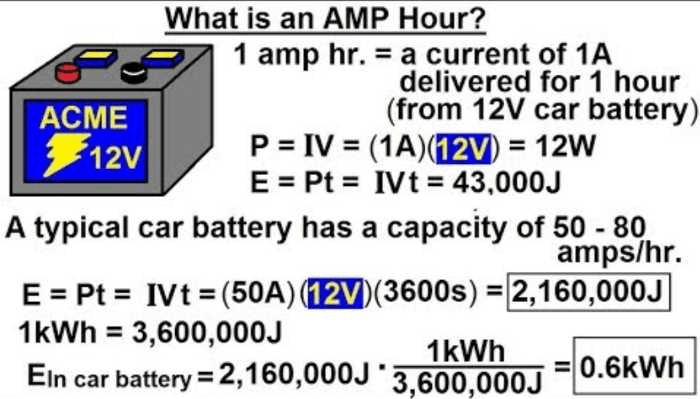When computing energy values in ampere hour, precise data on voltage is required. In a battery system, for example, precisely computing the provided energy necessitates integrating the power delivered (product of instantaneous voltage and instantaneous current) throughout the discharge period. However, as battery voltage generally varies during discharge, using an average or nominal value may be necessary to approximate the integration of power.

##### What is the formula for converting amp hours to watt hours?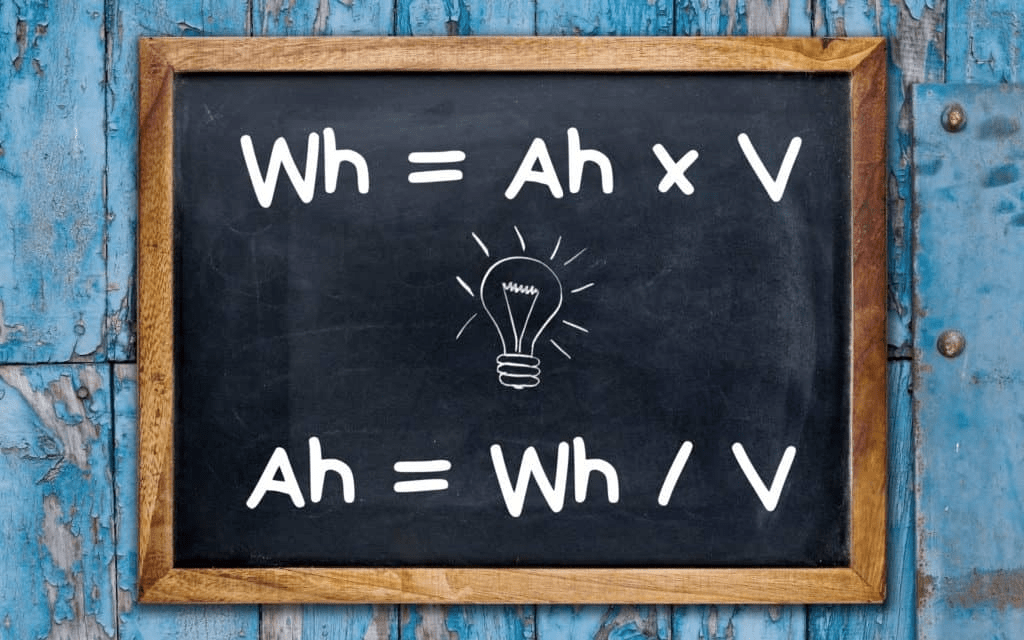From the example above, you can see how amp-hours do not equal energy. To get the watt-hours, you must multiply the total number of amp-hours by the voltage.

You can convert amp hours to watt-hours by using the equation:

watt-hours = amp-hours x volts.

For your RV, if you have a 12V battery and your device is rated for 100Ah, then you would need to multiply the amp hours (100Ah) by the volts (12V) to get 1200 watt-hours.

You could also get the same 1200 watt-hours from a 24V battery, but you would only need 50Ah since you multiply by 24V.

In other words, the number of volts will determine how many amp-hours you need to get the desired amount of watt-hours.

##### What is the Watt Hour capacity of a 100 Ah Lithium Battery?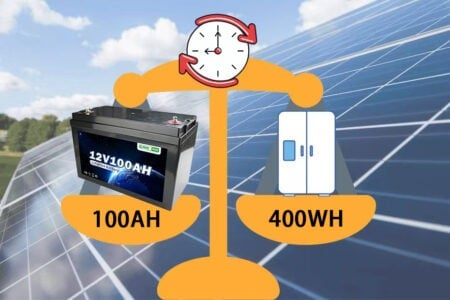You may be wondering how many watt-hours a 100 Ah lithium battery contains. To solve this question, we must first determine the voltage of the battery.Most 12V lithium batteries have a capacity of 1,200 watt-hours. This means the battery can provide 1,200 watts of power for one hour or 600 watts for two hours, etc.

The capacity of a lithium battery is usually measured in amp hours (Ah). One Ah is equal to 1,000 milliamp hours (mAh). This indicates that a 100 Ah battery can supply 100,000 mAh of electricity.

However, watt hours will vary slightly depending on the nominal voltage. For example, in a 12V Battle Born Battery, the voltage is a little over 13V. This means that the battery has a capacity of 1,300 watt-hours.

This difference is important to remember when comparing different battery types. For example, a lead-acid battery might have a higher Ah rating than a lithium battery, but the lithium battery will have a higher watt-hour rating, meaning it can provide more power.

Tom Morton of Mortons on the Move compares lead-acid and lithium batteries with watt-hour capacity in the video above. He discovers how difficult it is to obtain the claimed energy ratings from lead-acid batteries, as well as how much lithium batteries give under varied loads.

The video shows that the cost per watt-hour of electricity for one of our Battle Born Batteries is actually cheaper than that of all of the tested lead-acid rivals!

This makes lithium batteries a more cost-effective choice in the long run, even though they may have a higher initial price tag.

##### How long will the 100Ah battery last? 100W, 400W

Because 100Ah batteries can be used for RVs, solar panels, and cars, many people wonder about their battery life.

“How long will a 100 amp-hour battery last??”

The amount of power a motor consumes daily is determined by how many times it is run. This may be calculated using the formula below: When you understand the basic electric power law, it’s easy to figure out how many times a motor is utilized each day:

Power (W) = Current (I) × Voltage (V)

A 100Ah battery can power a 10W device for 120 hours or a 2,000W device for 36 minutes. This type of battery has a capacity of 1.2 kWh and is more than 2% as powerful as the Tesla Model 3 automobile battery.

Watt-hours are used to measure the juice in a 12V 100Ah battery. To know how long the battery will last, we need to look at how much capacity the appliance you’re running uses.

The 100-amp hour battery will power appliances for different amounts of time, as illustrated in the chart below: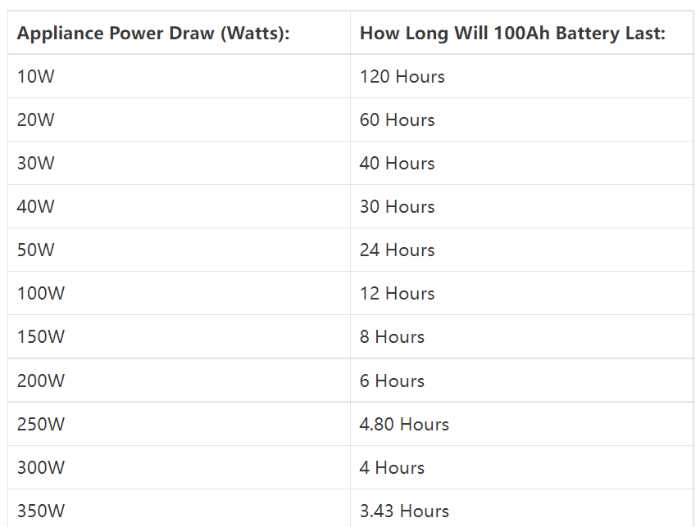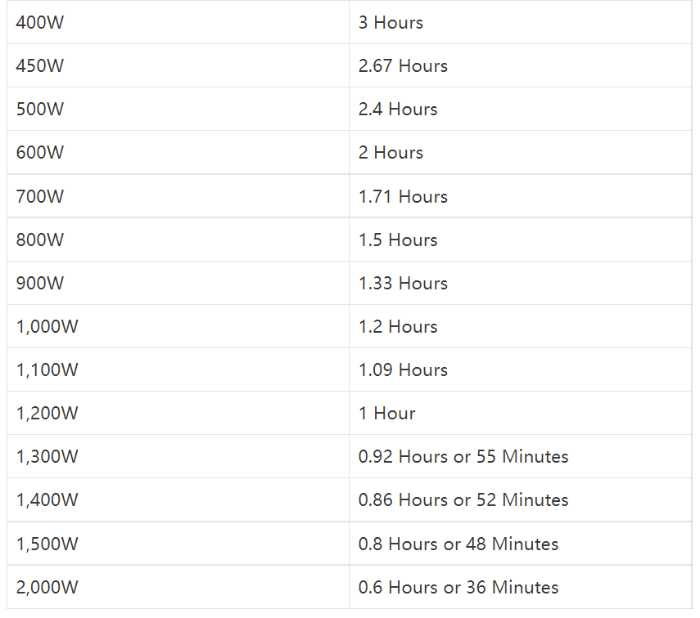Solving these examples will demonstrate how easy it is to calculate these times by hand.

##### A 100Ah battery will power a refrigerator that requires 400W for how long? (Example 1)
• Many people who own RVs or caravans are curious about how long a 100Ah battery would last if you ran a 400W appliance off it.

Trying to grasp a 100Ah just by looking at it might be difficult. However, if you multiply the 100Ah battery capacity by 1,200Wh, you can instantly determine how long the battery will last:

2. This appliance uses 400 watts of power.

To determine how long a 400W device will run on a 100Ah 12V battery, divide 1,200Wh by 400W. In a nutshell, a 100Ah 12V battery will power a 400W device for three hours.

##### Does the 100Ah battery keep an appliance powered up that consumes 100 watts for how long? (Example 2)
• We can use the same principle here. We know that a 100Ah battery will run a 100W appliance for 12 hours because we understand two metrics:

2. This appliance uses 100W.

When you convert the voltage to mV and divide it by 10,000mV, you get a result of 12h.

### Where Can I Find an Ah-Wh Conversion Table?

We know how confusing some amp-hour to watt-hour conversions can be, so we’ve put together this quick reference chart. Please remember that the watts per hour will change based on discharge and temperature changes.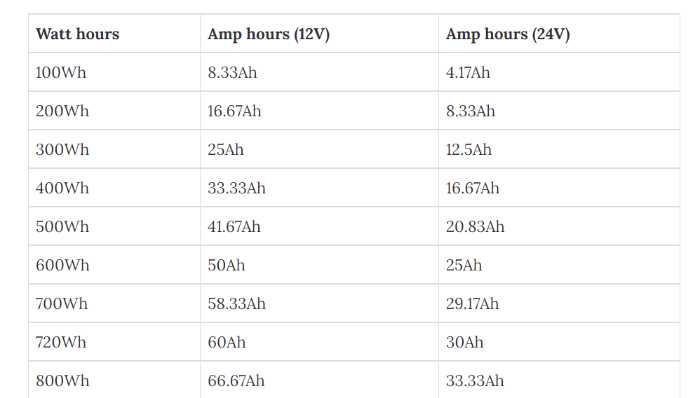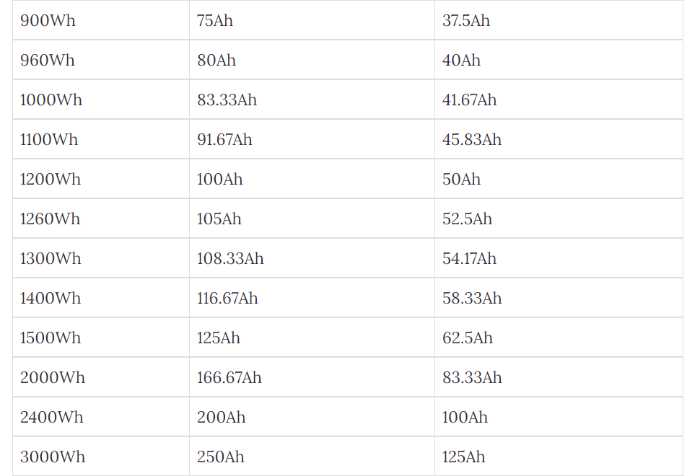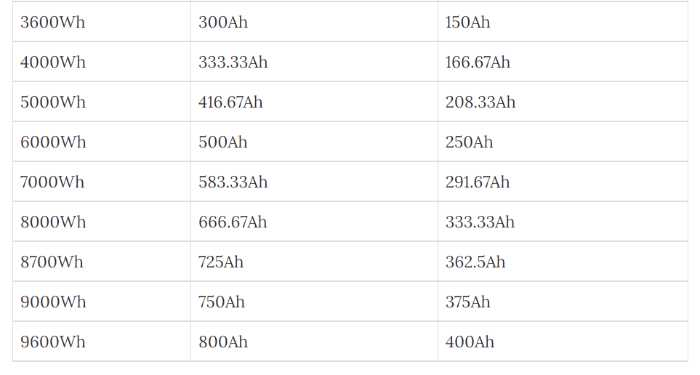The conversion calculators and graphs are free, but if you want the equations, Google is your friend. Knowing the equations will be beneficial if you need to calculate the amperage for your RV by adding together the watts of several gadgets. It’s never a bad idea that to have a firm grip on your battery’s power requirements, especially when you can’t check them online.

### When Will You Need to Calculate Watts and Amp Hours?

Watt- and amp-hour calculations are important to understand your battery’s energy capacity. This will come in handy for trying to run a device on battery power, as you’ll need to know how much battery capacity is required.

Simply put, watt-hours are equal to the number of watts multiplied by the number of hours of use. So, if you have a 50-watt item and want to use it for 10 hours, you’ll need 500 watt-hours.

Amp-hours are calculated by taking the total number of amps required for all devices and multiplying it by the number of hours of use. So, if you have two devices that each require 2 amps and you want to use them for 10 hours, you would need 20 amp-hours.

When designing your solar setup, you’ll need to consider the number of watt-hours required. To do this, add the watts used by each device and divide them by the voltage in your rig. This will provide you with the total amount of amp-hours required.

From there, you can determine the number and type of batteries needed and the size of the solar panels required. The thickness of connecting wires will also be determined by the amp-hours needed.

In short, watt- and amp-hour calculations are important to understand your battery’s energy capacity and how much power your devices use. This information is necessary when running devices on battery power or designing your solar setup.

### Knowledge Is PowerAs the saying goes, knowledge is power. And when it comes to batteries, that couldn’t be more true. You can effectively compare battery energy capacities by understanding how amp-hours and watt-hours are related. This knowledge is crucial when you need power off the grid. While many batteries are rated for “100 amp-hours,” you can now calculate how much energy such batteries will offer you and for how long.

With this knowledge, you’ll be able to select the best battery for your needs, whether it’s for charging your RV when camping off the grid or keeping your house operating during a power outage. So, when you are shopping for batteries, don’t only look at the amp-hour rating; also consider watt-hours. As a result, you’ll be able to make an educated conclusion.

If you are concerned about an electrical project, please do not hesitate to contact our staff. We at Green ONE Power would be delighted to give support and answer any queries you may have. You can reach out to us at (86) 191 2956 3884.